cancel
Showing results for
Did you mean:Helper II

## How to create table for first 3 month then Qtr 1, second 3 month Qtr 2 for

Hi Hello,

I have category and date. i need to create month wise sales like

:-   Apr, May,June Then Qtr 1, July, Aug, Sep, Than Qtr 2

Jan,Feb,March month then Qtr 4

1 ACCEPTED SOLUTIONCommunity Support

Hi @shri0025 ,

If you want to sort strictly by "month-quarter- month-quarter", you can try the following.
1. Create a new table and add Index column.

`````` Table.FromRecords({
[Name = "Apr-19", MonthNum = 4],
[Name = "May-19", MonthNum = 5],
[Name = "Jun-19", MonthNum = 6],
[Name = "Q1", MonthNum = null],
[Name = "Jul-19", MonthNum = 7],
[Name = "Aug-19", MonthNum = 8],
[Name = "Sep-19", MonthNum = 9],
[Name = "Q2", MonthNum = null],
[Name = "Oct-19", MonthNum = 10],
[Name = "Nov-19", MonthNum = 11],
[Name = "Dec-19", MonthNum = 12],
[Name = "Q3", MonthNum = null],
[Name = "Jan-19", MonthNum = 1],
[Name = "Feb-19", MonthNum = 2],
[Name = "Mar-19", MonthNum = 3],
[Name = "Q4", MonthNum = null]
})``````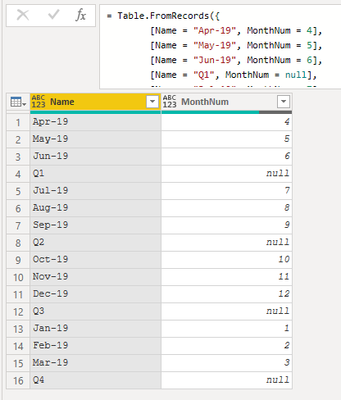2. Create the following measures.

``````sales =
CALCULATE(
SUM('Table'[Total Sales]),
FILTER(
'Table',
'Table'[Month Name] = MAX(AxisTable[Name])
)
)``````
``````Measure =
var QNo = VALUE( RIGHT( MAX(AxisTable[Name]), 1 ) )
var Q_M = If( QNo * 3 + 1 < 12, QNo * 3 + 1, 1 )
var Q_Sales =
CALCULATE(
SUMX(
ALLSELECTED(AxisTable[Name]),
[sales]
),
FILTER(
ALL(AxisTable),
AxisTable[MonthNum] in { Q_M, Q_M+1, Q_M+2 }
)
)
return
IF(
left( MAX(AxisTable[Name]), 1 ) = "Q",
Q_Sales,
[sales]
)``````

3. Sort the Name column by Index column and sort the bar chart by Name column in ascending order.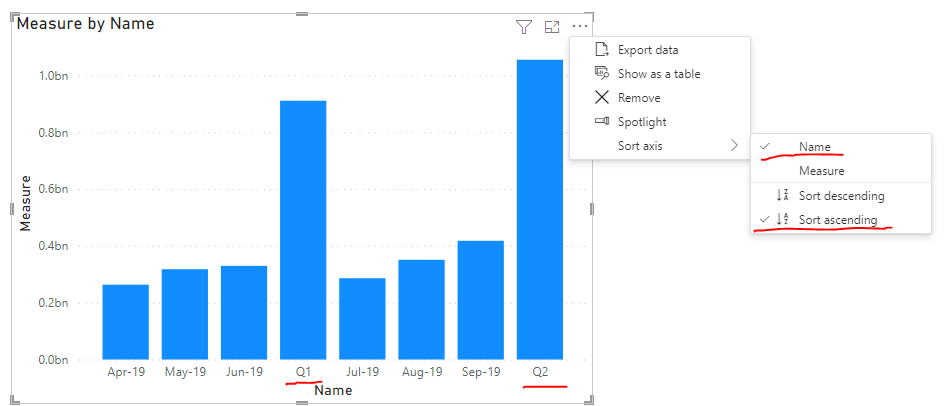If the problem is still not resolved, please provide detailed error information or the expected result you expect. Let me know immediately, looking forward to your reply.

Best Regards,
Winniz

If this post helps, then please consider Accept it as the solution to help the other members find it more quickly.

13 REPLIES 13Community Support

Hi @shri0025 ,

If you want to sort strictly by "month-quarter- month-quarter", you can try the following.
1. Create a new table and add Index column.

`````` Table.FromRecords({
[Name = "Apr-19", MonthNum = 4],
[Name = "May-19", MonthNum = 5],
[Name = "Jun-19", MonthNum = 6],
[Name = "Q1", MonthNum = null],
[Name = "Jul-19", MonthNum = 7],
[Name = "Aug-19", MonthNum = 8],
[Name = "Sep-19", MonthNum = 9],
[Name = "Q2", MonthNum = null],
[Name = "Oct-19", MonthNum = 10],
[Name = "Nov-19", MonthNum = 11],
[Name = "Dec-19", MonthNum = 12],
[Name = "Q3", MonthNum = null],
[Name = "Jan-19", MonthNum = 1],
[Name = "Feb-19", MonthNum = 2],
[Name = "Mar-19", MonthNum = 3],
[Name = "Q4", MonthNum = null]
})``````2. Create the following measures.

``````sales =
CALCULATE(
SUM('Table'[Total Sales]),
FILTER(
'Table',
'Table'[Month Name] = MAX(AxisTable[Name])
)
)``````
``````Measure =
var QNo = VALUE( RIGHT( MAX(AxisTable[Name]), 1 ) )
var Q_M = If( QNo * 3 + 1 < 12, QNo * 3 + 1, 1 )
var Q_Sales =
CALCULATE(
SUMX(
ALLSELECTED(AxisTable[Name]),
[sales]
),
FILTER(
ALL(AxisTable),
AxisTable[MonthNum] in { Q_M, Q_M+1, Q_M+2 }
)
)
return
IF(
left( MAX(AxisTable[Name]), 1 ) = "Q",
Q_Sales,
[sales]
)``````

3. Sort the Name column by Index column and sort the bar chart by Name column in ascending order.If the problem is still not resolved, please provide detailed error information or the expected result you expect. Let me know immediately, looking forward to your reply.

Best Regards,
Winniz

If this post helps, then please consider Accept it as the solution to help the other members find it more quickly.Solution Sage

So you want to show in one visual the data for each month as well as the total for each quarter?Helper II

YesSolution SageHelper II

Hi Please find input data show to Visual bar Chart

 Month Name Total Sales Apr-19 263417035 May-19 317719685 Jun-19 329506493 Q1 910643213 Jul-19 286064978 Aug-19 350989685 Sep-19 417868113 Q2 1054922777Solution Sage

Are you sure this is what your data looks like when it is imported into PowerBi?

In that case you can go to PowerQuery and add an index column:Then go back to the report and select the column Month Name --> Sort by column: Index

Put Month Name and Total Sales in the Column chart and sort by Month:If I answered your question, please mark it as a solution to help other members find it more quickly.Helper II

Hi Thanks For Help

I had mentioned Q1,Q2 only for reference. Otherwise My data only has month wise sales.

I want to add QTR bar as well after 3 months in the BAR CHART.Solution Sage

@shri0025 In that case, can you please share the data how you import it in PowerBi?

Is it like this?

 Month Name Total Sales Apr-19 263417035 May-19 317719685 Jun-19 329506493 Jul-19 286064978 Aug-19 350989685 Sep-19 417868113Helper II

Hi

Yes Look like the SameSolution Sage

I'm not sure if this is the easiest solution, but it worked for me:

Go to the Query Editor and add a column for the Qtr: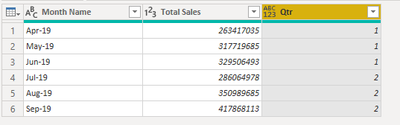Duplicate the table twice, in one table you keep only the Month Name column and in the other the Qtr Column: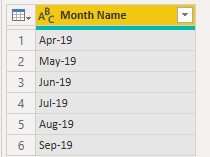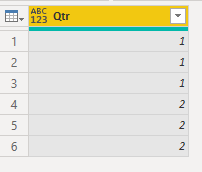Remove the duplicates in the Qtr column and change the name to Month Name

Then append the last table to the second table and add an index column: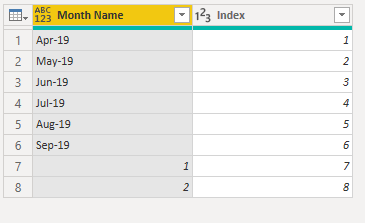Close & apply and go to relationships. Create two relationships between the tables:
Table - Month Name to Table (2) - Month Name and Table - Qtr to Table (2) - Month Name  (inactive).Then create this measure:

Measure =
var Sales = SUM('Table'[Total Sales])
return
IF(ISBLANK(Sales),CALCULATE(SUM('Table'[Total Sales]),USERELATIONSHIP('Table'[Qtr],'Table (2)'[Month Name])),Sales)

Put the measure and Month Name column from table 2 in the visual to get this result:Hope this helps!

If I answered your question, please mark it as a solution to help other members find it more quickly.Helper IISolution Sage

Hi @shri0025 ,

What does your input data look like?

And what is the expected result?Helper IILook Like Bellow